Next:Properties of Shannon's EntropyUp:Shannon's Entropy

# Measures of Uncertainty: Shannon's Entropy

Let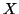be a discrete random variable taking a finite number of possible values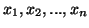with probabilities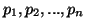respectively such that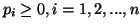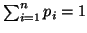. We attempt to arrive at a number that will measure the amount of uncertainty. Let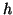be a function defined on the interval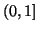and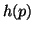be interpreted as the uncertainty associated with the event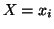,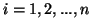or the information conveyed by revealing thathas taken on the value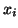in a given performance of the experiment. For each n, we shall define a function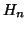of the n variables. The function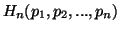is to be interpreted as the average uncertainty associated with the event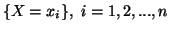given by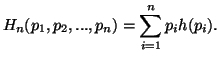(1.1)

Thusis the average uncertainty removed by revealing the value of. For simplicity we shall denote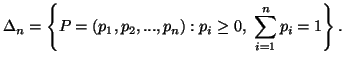We shall now present some axiomatic characterizations of the measure of uncertainty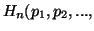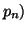to arrive at its exact expression. For that, letand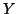be two independent experiments with n and m values respectively. Let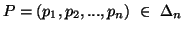be a probability distribution associated withand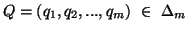be a probability distribution associated with. This lead us to write that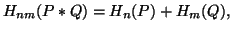(1.2)

for all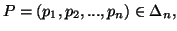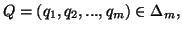and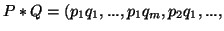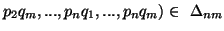. Replacing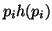by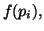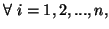we get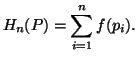(1.3)

Based on (1.2) and (1.3) we present the following theorem.

Theorem 1.1. Let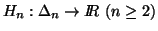be a function satisfying (1.2) and (1.3), where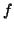is real valued continuous function defined over [0,1]. Thenis given by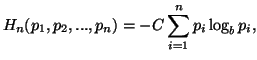(1.4)

where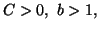with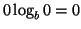.

The proof is based on the following Lemma (ref. Chaundy and McLeod, 1961 ).

Lemma 1.1. Let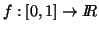be a continuous function satisfying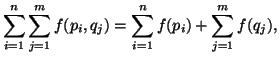(1.5)

for all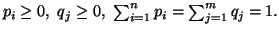Then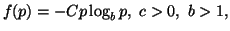(1.6)

for all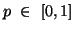with.

Alternatively the measure (1.4) can be characterized as follows (ref. Shannon,1948 ; Feinstein, 1958 ).

Theorem 1.2. Letbe a function satisfying the following axioms:

(i)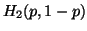is a continuous function of.
(ii)be a symmetric function of its arguments.
(iii)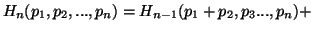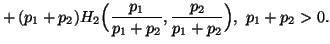Thenis given by (1.4).

A third way to characterize the measure (1.4) is as follows (ref. Aczél and Daróczy, 1975 ).

Theorem 1.3. Let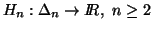be a function satisfying the following axioms:

(i)is a continuous and symmetric function with respect to its arguments.
(ii)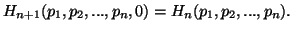(iii)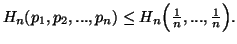(iv) For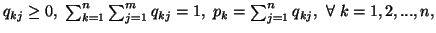we have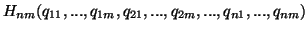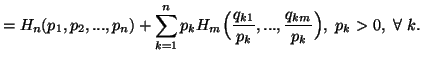Thenis given by (1.4).

The following is a different way to characterize the measure (1.4). It is based on the functional equation famous as fundamental equation of information.

Theorem 1.4. Letbe a function satisfying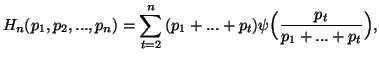where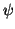satisfies the following functional equation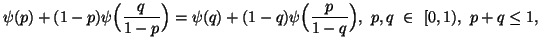with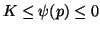for all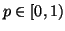. Thenis given by (1.4).

For simplicity, let us take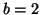in (1.4). If we put the restriction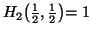in the above theorems with, we get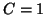. This yields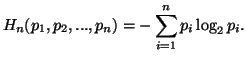(1.7)
The expression (1.7) is famous as Shannon's entropy or measure of uncertainty.

For more characterizations of the measure (1.4) or (1.7) refer to Aczél and Daróczy (1975)  and Mathai and Rathie (1975) .

21-06-2001
Inder Jeet Taneja
Departamento de Matemática - UFSC
88.040-900 Florianópolis, SC - Brazil